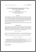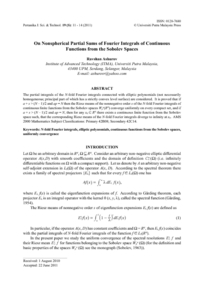Simple Search:

# On nonspherical partial sums of fourier integrals of continuous functions from the Sobolev spaces

## Citation

Ashurov, Ravshan (2011) On nonspherical partial sums of fourier integrals of continuous functions from the Sobolev spaces. Pertanika Journal of Science & Technology, 19 (S). pp. 11-14. ISSN 0128-7680; ESSN: 2231-8526

## Abstract / Synopsis

The partial integrals of the N-fold Fourier integrals connected with elliptic polynomials (not necessarily homogeneous; principal part of which has a strictly convex level surface) are considered. It is proved that if a + s > (N – 1)/2 and ap = N then the Riesz means of the nonnegative orders of the N-fold Fourier integrals of continuous finite functions from the Sobolev spaces Wpa(RN) converge uniformly on every compact set, and if a + s > (N – 1)/2 and ap = N, then for any x0∈ RN there exists a continuous finite function from the Sobolev space such, that the corresponding Riesz means of the N-fold Fourier integrals diverge to infinity at x0. AMS 2000 Mathematics Subject Classifications: Primary 42B08; Secondary 42C14Preview
PDF
On Nonspherical Partial Sums of Fourier Integrals of Continuous.pdfView Item